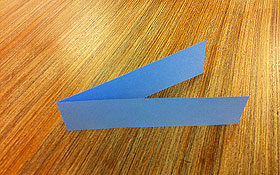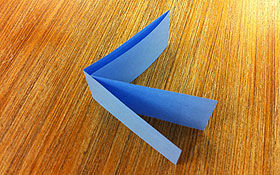Home > Patterns > Activities > Folding patterns

# Folding patterns

Take a 30 cm long strip of paper and fold it in two.The strip folded in two.

Then unfold it and ask students: How many parts are there?

Point out that the two parts are the same size (because they match) so that each part is a half of the strip. Also count the number of folds.

Fold the strip again and then fold it in two a second time.The strip folded in two, twice.

Ask the students to predict the number of parts and folds.

Unfold the strip and check that there are four equal parts and three folds.

Ask the students to identify quarters.

Repeat this process at least twice more and make a table like this.

 No. of times folded No. of equal parts Name of each part No. of folds 1 2 half 1 2 4 quarter 3 3 4

Now ask students to describe and explain the patterns in the table. You can download Patterns in the Table which gives a completed table and some discussion points.

Note especially the doubling pattern in the second column and the way in which unitary fractions are named.

Able students could be asked to predict the 10th row of the table.

See Growing fractions for another pattern activity involving fractions.